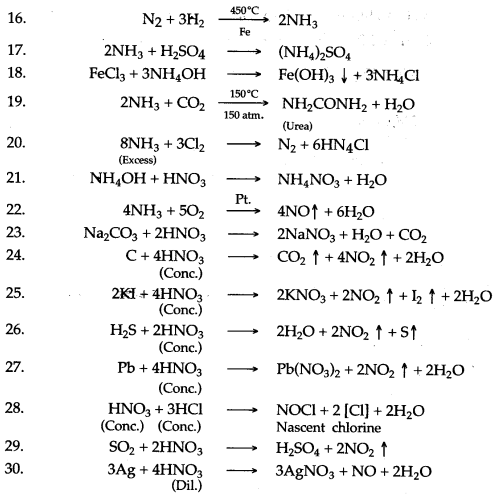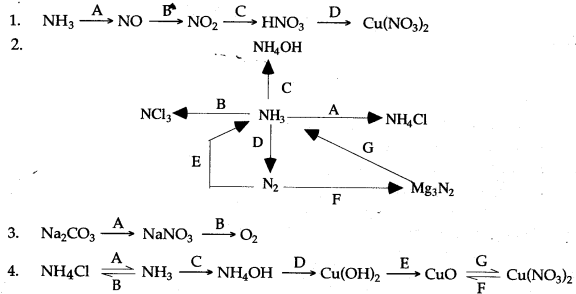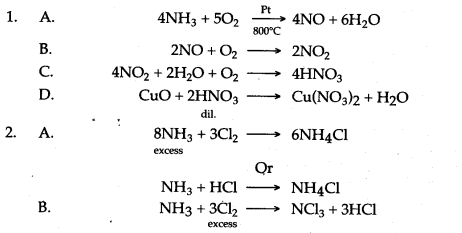ICSESolutions.com provides ICSE Solutions for Class 10 Chemistry Chapter 9 Study of Compounds: Ammonia and Nitric Acid for ICSE Board Examinations. We provide step by step Solutions for ICSE Chemistry Class 10 Solutions Pdf. You can download the Class 10 Chemistry ICSE Textbook Solutions with Free PDF download option.

## ICSE Class 10 Chemistry Chapter 9 Study of Compounds: Ammonia and Nitric Acid Solutions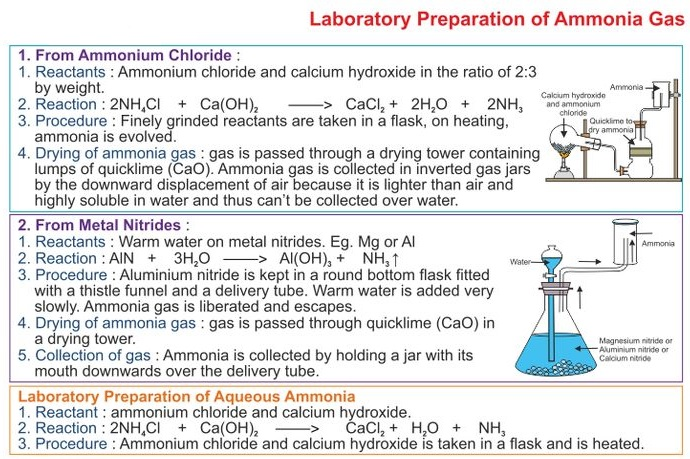### Short Questions

Question 1: How liquor ammonia is different from liquid ammonia ?
Answer: A very strong solution of ammonia in water is called liquor ammonia. Ammonia can be obtained from it by boiling. When ammoniacal liquor is boiled with milk of lime, the ammonia formed is passed into ice or cold water to form liquor ammonia.
When cooled under pressure, ammonia condenses to a colourless liquid which boils at – 33.4°C. This is liquid ammonia which when further cooled freezes to a white crystalline snow like solid, which melts at – 77.7°C. Liquid ammonia is used as a refrigerant because its heat of vaporisation is 5700 calories per gram molecule. Liquid ammonia is liquified ammonia and is available in cylinders. Laboratory bench reagent is a diluted solution of liquor ammonia.

Question 2: The questions below are related to the manufacture of ammonia.
(i) Name the process.
(ii) In what ratio must the reactants be taken ?
(iii) Name the catalyst used.
(iv) Give the equation for the manufacture of ammonia.
(v) Ammonia can act as a reducing agent — write a relevant equation for such a reaction.
(ii) Nitrogen one part, hydrogen three parts.
(iii) Iron powder.Question 3: The following questions are based on the preparation of ammonia gas in the laboratory :
(i) Explain why ammonium nitrate is not used in the preparation of ammonia.
(ii) Name the compound normally used as a drying agent during the process.
(iii) How is ammonia gas collected ?
(iv) Explain why it is not collected over water.
(v) Give the name of a hydride of nitrogen.
(vi) Which reactants are used in laboratory preparation of ammonia ?
(vii) What is the vapour density and nature of ammonia ?
(viii) Which feature of the Ammonia molecules leads to the formation of the Ammonium ion when Ammonia dissolves in water. Name the other ion formed when Ammonia dissolves in water.
Answer: (i) Because ammonium nitrate is explosive in nature and dissociate into nitrous oxide and water on heating.
(ii) Quick lime.
(iii) By downward displacement of air.
(iv) It is highly soluble in water.
(v) Ammonia.
(vi) Ammonium chloride and slaked lime [Ca(OH)2].
(vii) Vapour density of ammonia is 8.5, and it is alkaline in nature.
(viii) In ammonia molecule there is one lone pair of electrons available on nitrogen atom. This lone pair of electron leads to the formation of ammonium ion. Hydroxide ion (OH).

Question 4: What are the necessary conditions for getting maximum yield of ammonia ?
Answer: The necessary conditions for getting maximum yield of ammonia are as follows :
(i) Low Temperature : As the reaction is exothermic in nature, so the temperature should be low. When temperature is lowered, the rate of the reaction slows down and the yield is maximum. It is found for maximum yield, temperature should be between 450° to 500°C (optimum temperature).
(ii) Catalyst: A catalyst is used to accelerate the reaction at optimum temperature. Following
are the catalysts used:
(a) Finely divided iron, mixed with molybdenum as promoter.
(b) A better catalyst is ferric oxide (Fe2O3) containing 1% of potassium oxide (K2O) and 3% of aluminium oxide (Al2O3) which acts as promoters.
(iii) High Pressure : When 4 volumes of a mixture of nitrogen and hydrogen are reduced to 2 volumes of ammonia, the pressure drops. In order to have maximum yield the pressure should be very high. The optimum pressure should be between 200 atmospheres to 900 atmospheres.

 Pressure (in atm.) Temperature (in °C) Yield (in %) 200 atms. 400°C 36.3% 1000 atms. 400°C 79.8%

(iv) Purity of Gases : Any kind of impurity tends to slow down the reaction or poisons catalyst. So, the gases that are to be used should be pure and dry.

Question 5: (i) What are the products formed when ammonia is oxidised with copper oxide ?
(ii) What is the difference between chemical nature of an aqueous solution of hydrogen chloride and an aqueous solution of ammonia. .
Answer: (i) Nitrogen, copper and water.
(ii) Aqueous solution of HCl is acidic while aqueous solution of ammonia is basic.

Question 6: How would you prepay :
(i) Nitrogen from ammonia. (ii) Ammonia from nitrogen.
(iii) Nitric oxide gas and nitrous oxide gas.
Answer: (i) Ammonia gas bums in the presence of oxygen in a glass jar with a greenish yellow flame to form nitrogen and steam.
4NH3 + 3O2  ⟶  2N2 + 6H2O
(ii) Nitrogen combines with heated metals; for example, aluminium to form aluminium nitride, which is readily decomposed by water to form ammonia gas which has a characteristic pungent odour and aluminium hydroxide is also formed.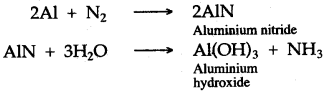(iii) Introduce the given gas in the atmosphere of oxygen and if reddish brown fumes are produced, then the given sample of gas is nitric oxide and if no brown fumes are produced and the mixture remains colourless, then the gas is nitrous oxide.

Question 7: Outline the conditions of the synthetic process for producing ammonia gas. Mention the catalyst used.
Answer: A mixture of nitrogen and hydrogen in the ratio of 1 : 3 by volume is passed over heated iron at 450°C in the presence of molybdenum, which acts as catalyst (promoter) under 200 atmospheric pressure. The reaction of nitrogen and hydrogen is a reversible reaction hence ammonia is removed from the reaction vessel from time to time by liquefaction under high pressure, so that the reaction proceeds in the forward direction.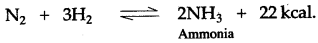Question 8: Name one element in each case, to which the following description would apply.
(i) The burning metal which combines directly with nitrogen.
(ii) Define fixation of nitrogen and state two ways in which fixation of nitrogen occurs, naturally.
(iii) Give two reasons to show that the solution of ammonia in water contains hydroxide ions.
Answer: (i) Magnesium, calcium and aluminium.
(ii) Conversion of atmospheric nitrogen into soluble nitrogenous Compounds which can be easily absorbed by the roots of the plants.
(a) During thunder and lightning
(b) By symbiotic bacteria
(iii) (a) It turns red litmus solution to blue.
(b) It reacts with adds to form salt and water.

Question 9: (i) Of the two gases, ammonia and hydrogen chloride, which is more dense ? Name the method of collection of this gas.
(ii) Give one example of a reaction between the above two gases which produces a solid compound.
Answer: (i) HCl collected by upward displacement of air
(ii) NH3 + HCl  ⟶  NH4Cl

Question 10: (i) Does ammonia bum in oxygen ? If yes, give equation.
(ii) What is catalytic oxidation of ammonia ?
(iii) What is the special feature of the apparatus that is used in the laboratory preparation of nitric acid ?
(iv) Why should the temperature of the reaction mixture of nitric acid not be allowed to rise above 200°C ?
Answer: (i) Yes. Ammonia bums in oxygen with a greenish yellow flame producing water vapour and nitrogen.
4NH3 + 3O2  ⟶  2N2 + 6H2O
(ii) Ammonia, when reacts with oxygen in presence of platinum (as catalyst) at 800°C, ammonia is oxidised to nitric oxide (NO). This is called catalytic oxidation of ammonia.
(iii) It is an all glass retort.
(iv) Because above 200°C nitric acid will decompose.

Question 11: Oxidation of ammonia under certain conditions is represented by the following equation.
4NH3 + 5O2  ⟶  4NO + 6H2O
(i) Give the conditions required for this reaction.
(ii) Briefly describe the importance of this reaction in industry.
Answer: (i) Pure and dry ammonia gas mixed with air in the ratio of 1 : 8 by volume is first compressed and then passed over heated platinum gauze at 800°C.
(ii) Nitric oxide is obtained by the oxidation of ammonia, which is further oxidised to form nitrogen dioxide. Nitrogen dioxide is dissolved in water, in the presence of oxygen, to form nitric acid. Thus, nitric acid can be prepared by the catalytic oxidation of ammonia.Question 12: (i) What would you see during burning of ammonia in oxygen.
(ii) Name the catalyst used in the catalytic oxidation of ammonia.
(iii) In the reaction of the catalytic oxidation of ammonia the catalyst glows red hot, Why ?
(iv) What is the name of the industrial process, which starts with the reaction of catalytic oxidation of ammonia.
(v) How is the temperature maintained in the Ostwald’s process ?
Answer: (i) The bunting of ammonia in oxygen produces a bluish green flame. The reaction is irreversible and highly exothermic.
(ii) Platinum.
(iii) The catalyst glows red hot without external heating because much heat is liberated during the reaction.
(iv) Ostwald’s process.
(v) In the Ostwald’s process, the catalytic oxidation of ammonia to nitric oxide is an exothermic reaction. Once the reaction starts, the heat released maintains the temperature of the catalyst.

Question 13: (i) How would you obtain the compound magnesium nitride ?
(ii) What property of ammonia is illustrated by the reaction between ammonia and copper oxide.
(iii) What important industrial process starts with reaction between ammonia and oxygen. Name the catalyst uses.
(iv) During laboratory preparation how is ammonia dried and collected ?
Answer: (i) By burning magnesium in the atmosphere of nitrogen by direct combination of elements.
3Mg + N2  ⟶  Mg3N2
(ii) Reducing property of Ammonia, as it reduces CuO to Cu in reaction.
(iii) Ostwald process for the manufacture of nitric acid and the catalyst used is platinum gauze.
(iv) Ammonia is dried by passing it through quick lime (CaO) and is collected by downward displacement of air.

Question 14: (i) Give the balanced equations for laboratory preparation of nitric acid and describe the reaction.
(ii) In laboratory preparation of nitric acid, the mixture of potassium nitrate and concentrated sulphuric add should not be heated above 200°C. Explain ?
Answer: (i) In laboratory, nitric acid is prepared by heating potassium nitrate with concentrated sulphuric acid at low temperature, potassium hydrogen sulphate and nitric acid are formed, and at a higher temperature, potassium sulphate and nitric add are formed.
Low temp.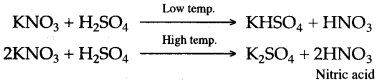(ii) In laboratory preparation of nitric acid, the mixture of potassium nitrate and concentrated sulphuric acid should not be heated above 200°C because at a higher temperature, potassium sulphate is formed. Potassium sulphate is deposited as a hard, solid mass in the retort, which is difficult to remove. Moreover, most of the nitric acid decomposes at a higher temperature to form reddish brown fumes of nitrogen dioxide and the acid acquire a yellow colouration.

Question 15: (i) (a) What compounds are required for the laboratory preparation of nitric acid ?
(b) The first step in the manufacture of nitric acid is the catalytic oxidation of ammonia. What is the name of the catalyst ?
(ii) (a) How soluble is ammonia in water ?
(b) Give two reasons to show that the solution of ammonia in water contains hydroxide ions.
(c) Name a simple method you would employ to prepare ammonium salts in your laboratory.
Answer: (i) (a) Nitre (Potassium Nitrate) and cone, sulphuric acid.
(b) Platinum.
(ii) (a) Extremely soluble.
(b) Ammonia solution in water turns red litmus blue and phenolphthalein pink. Ferric chloride, aluminium chloride solutions treated with ammonia solution give precipitates of Fe(OH)3 and Al(OH)3 respectively.(c) By titration or neutralisation.

Question 16: (i) Among the elements chlorine, nitrogen and sulphur select:
(a) The least reactive. (b) Obtained from the atmosphere.
(ii) Why commercial nitric acid is brown ?
(iii) How can you get ‘liquid air’ ?
Answer: (i) (a) Nitrogen. (b) Nitrogen.
(ii) Commercial nitric acid is brown in colour because it contains dissolved nitrogen dioxide.
(iii) CO2 and moisture-free air is passed through a condenser. Finally, this air is compressed, cooled and suddenly allowed to expand. By repeating this process, the air can be liquified.

Question 17: Give two reactions to show that nitric acid is :
(i) An acid.
(ii) An oxidizing agent.
Answer: (i) (a) Dilute nitric acid reacts with magnesium metal to liberate hydrogen gas and magnesium nitrate is formed.
Mg + 2NHO3  ⟶  Mg(NO3)2 + H2
(b) Dilute nitric acid reacts with marble pieces to liberate carbon dioxide gas and calcium nitrate is formed.
CaCO3 + 2HNO3  ⟶  Ca(NO3)2 + H2O + CO2
(ii) (a) When carbon is heated with concentrated nitric acid, it is oxidized to carbonic add and the acid is reduced to nitrogen dioxide and water.
C + 4HNO3  ⟶  H2CO3 + 4NO2 + H2O
(b) When sulphur is heated with concentrated nitric acid, it is oxidized to sulphuric acid and the nitric’add is reduced to nitrogen dioxide and water. Carbonic add further decomposes to carbon dioxide.
S + 6HNO3 (Conc)  ⟶  H2SO4 + 6NO2 + 2H2O

Question 18: In what way does the action of dilute nitric acid on a metal like zinc differ from the action of dil. sulphuric acid. Or
Dilute Nitric acid is generally considered a typical add except for its reaction with metals. In what way is dilute Nitric acid different from other acids when it reacts with metals ?
Answer: Nitric add is a strong oxidising agent and oxidises the liberated hydrogen to water and is itself reduced to nitrogen dioxide. On the other hand dilute sulphuric acid can not oxidise the liberated hydrogen to water.

Question 19: The reaction of nitric acid with metals depends upon the concentration of the acid. Give examples, alongwith equations to illustrate the reaction of the add.
(i) Copper reacts with cold and dilute nitric add to form copper nitrate, water and nitric oxide gas is liberated.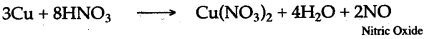(ii) Copper reacts with hot and concentrated nitric acid to form copper nitrate, water and nitrogen dioxide.gas, which has reddish brown fumes and a pungent smell is liberated.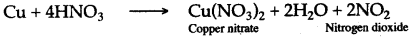Question 20: Answer the questions given below, relating your answer only to compounds given in the following list:
Tetrammine copper (II) sulphate, Iron (III) chloride, cone. Nitric add, Ammonium hydroxide.
(i) Write name of the colours and action when concentrated nitric acid is heated with copper turnings ?
(ii) A compound X is dissolved in the ammonia solution to give a deep blue coloured solution, write the name of the compound having deep blue colours ?
(iii) The concentrated nitric acid produces yellow stains and blisters on skin. Give the reason.
Answer: (i) Brown fumes of nitrogen dioxide are evolved.
(ii) Tetrammine copper (II) sulphate.
(iii) This is due to the chemical reaction that occurs between nitric acid and proteins of skin and flesh. In this reaction, a complex compound xanthoprotic acid is formed.

Question 21: The action of heat on the blue crystalline solid L gives a reddish brown gas M, a gas which relights a glowing splint and leaves a black residue. When as N, which has a rotten egg smell, is passed through a solution of L a black precipitate is formed.
(i) Identify L, M and N (Name or formula).
(ii) Write the equation for the action of heat on L.
(iii) Write the equation for the reaction between the solution of L and the gas N.
Answer: (i) L is copper nitrate.
M is nitrogen dioxide gas.
N is hydrogen sulphide gas.Question 22: How will you show that nitric arid contains :
(i) Hydrogen (ii) Nitrogen (iii) Oxygen ?
Answer: (i) Dilute nitric acid reacts with magnesium metal to liberate hydrogen gas which bums with a pale blue flame and a pop sound and magnesium nitrate is formed.
Mg + 2HNO3 (Dil)   ⟶  Mg(NO3)2 + H2
(ii) Nitric arid when heated with copper turnings, produces brown fumes of nitrogen dioxide, which are passed over red hot iron filings. The residual gas collected over water is nitrogen.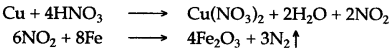(iii) Nitric arid, when heated alone, is decomposed into a reddish brown coloured gas nitrogen dioxide, steam and a colourless, odourless gas oxygen, which rekindles a glowing splinter.
4HNO3   ⟶  4NO2 + 2H2O + O2

Question 23: What is the ring test for nitric acid ? Briefly describe this test.
Answer: To the nitrate solution, add freshly prepared iron (II) sulphate solution. Filter off the precipitate if formed. Now carefully add cone. H2SO4 along the sides of the test tube. A dark , brown ring is formed at junctions of two liquids. Brown ring is due to the formation of
nitrosonium pentaqua iron (I) sulphate [Fe+ (NO+) (H2O)5] SO4, which is an octahedral complex compound.

Question 24: Gas B turns moist red litmus paper blue.
(i) What is the name of gas B ?
(ii) Write the equation for the reaction that takes place when gas B is passed over- heated copper oxide.
Answer: (i) Gas B is ammonia.
(ii) 3CuO + 2NH3    3Cu + 3H2O + N2

Question 25: (i) Sodium hydroxide solution is added to solution A, a white precipitate is formed which is soluble in excess of sodium hydroxide. What metal ion is present in A ?
(ii) Ammonium hydroxide solution is added to solution B, when a pale blue precipitate is formed. This pale blue precipitate dissolves in excess of ammonium hydroxide to give inky blue colouration. Name the cation present in B. Name the probable colour of solution B.
(iii) When an ammonium salt is warmed with sodium hydroxide solution, ammonia gas is evolved. State three ways in which you can identify the gas.
Answer: (i) Ammonium ion is present in A.
(ii) The cation present in B is copper. Solution B is blue in colour.
(iii) The three ways in which the gas can be identified are as follows :
(a) It gives dense white fumes when a rod dipped in HCl is held in it.
(b) It turns moist red litmus paper blue.
(c) It turns phenolphthalein solution pink.

### Figure/Table Based Questions

Question 1: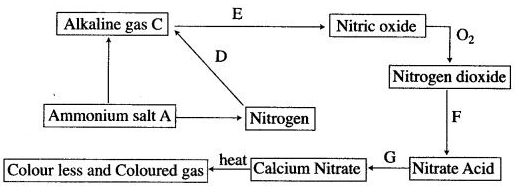(i) Name (a) the ammonium salt A (b) alkaline gas C.
(ii) How the conversion D is carried out ? State all the conditions like temperature, pressure and catalyst.
(iii) (a) How is C converted to nitric oxide ? Write the equation.
(b) What is the name of the process ?
(c) How is temperature maintained in above process ?
(d) Write the equation for conversion of F and G.
(iv) Name the coloured gas and the colourless gas.
Answer: (i) (a) Ammonium nitrate, NH4NO3
(b) Ammonia.
(ii) D is mixed with hydrogen in the ratio of 1 : 3, compressed to a pressure of 200 to 500 atmosphere and passes over a catalyst (iron) heated to 450 to 500°C.
(iii) (a) By passing the gas with excess of air over platinum gauze heated to 800°C.
4NH3 + 5O2  ⟶  4NO + 6H2O
(b) Ostwald process.
(c) The oxidation of ammonia to nitric oxide is exothermic reaction and once the reaction is started it maintains the temperature of the platinum gauze.(iv) Nitrogen dioxide and oxygen.

Question 2: The diagram shows an experimental set up for the laboratory preparation of a pungent smelling gas. The gas is alkaline in nature.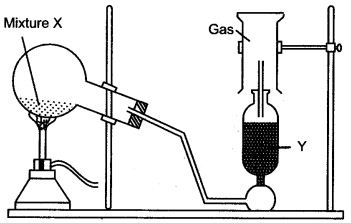(i) Name the gas collected in the jar.
(ii) Write the balanced equation for the above preparation.
(iii) How is the gas being collected ?
(iv) Name the drying agent used.
(v) How will you find that the jar is full of gas ?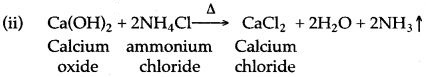(iii) By downward displacement of air.
(iv) Quick lime (CaO).
(v) By bringing a wet red litmus paper near the brim (mouth) of the gas jar. When jar is full of gas litmus will turn blue.

Question 3: Dry ammonia gas is passed over black substance as shown in figure below :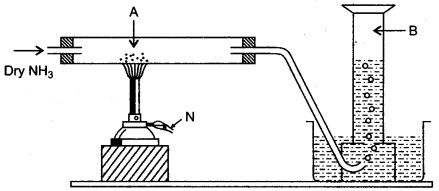(i) Name the black substance A.
(ii) Name the gas evolved B.
(iii) Write a balanced equation for the reaction of ammonia with A.
(iv) What do you observe when ammonia is passed over copper oxide ?
(v) State the property illustrated in the above reaction (iii).
(ii) Nitrogen gas.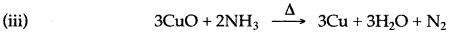(iv) The black mass changes t0 reddish powdery mass.
(v) Ammonia is a reducing agent.

Question 4: The figure given below illustrates the apparatus used in the laboratory preparation of nitric acid.(i) Name A (a liquid), B (a solid) and C (a liquid). (Do not give the formulae)
(ii) Write an equation to show how nitric acid undergoes decomposition.
(iii) Write the equation for the reaction in which copper is oxidized by concentrated nitric acid.
Answer: (i) A-Conc. Sulphuric acid, B-Potassium nitrate or Sodium nitrate, C-Nitric acidQuestion 5: (i) Draw a neat sketch for.the manufacture of ammonia by Haber’s process.
(ii) Discuss the principles involved in Haber’s process.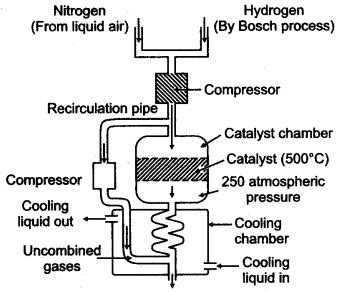(ii) The principles involved in Haber’s Process are :
In this process, a mixture of pure, dry nitrogen and hydrogen in the ratio of 1 : 3 is compressed to a pressure of 200 to 900 atmospheres. The compressed gases are passed over heated catalyst (finally divided molybdenum, i.e. Fe/Mo) at 450° to 500°C.The above reaction is exothermic and reversible. It is accompanied by decrease in volume. Ammonia formed is immediately removed from the catalytic chamber and allowed to condense to form liquid ammonia.

Question 6: Study the scheme for dilute nitric acid.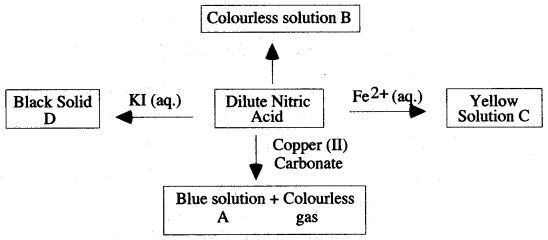(i) Give the name or formula of
(a) Solution A
(b) Solution B
(c) Acation in solution C
(d) Solid D
(ii) What property of nitric acid is shown by its reaction with iron (II) ions and aqueous potassium iodide solution ?
(iii) Describe one other reaction of dilute nitric acid not shown in the reaction scheme, that is typical of a strong acid.
Answer: (i) (a) Copper (II) nitrate Cu(NO3)2
(b) Ammonium nitrate
(c) Fe3+
(d) Iodine
(ii) Oxidising property of nitric acid.
(iii) It is a characteristic of strong acids that when dilute, they react with the more electropositive metals, liberating hydrogen gas.
Zn + 2H+ ⟶  Zn2+ + H2
This reaction can not occur with nitric acid as it is a powerful oxidising agent. Any hydrogen initially produced is at once oxidised by more of the acid to water. If however very dilute nitric acid is used (about 1%) with magnesium or manganese, some hydrogen will be produced, escaping oxidation because of the very dilute condition of the acid.
Mg + 2HNO3 (very dil.)  ⟶  Mg(NO3)2 + H2

Question 7: Copy and complete the following table relating to the important industrial process. Output refers to the product of the process and not the intermediate steps.

 Name of process Inputs Catalyst Equation for catalyst reaction Output Ammonia + air Nitric addQuestion 8: Copy and complete the following table relating to important industrial process:

 Name of the process Temperature Catalyst Equation for the catalyzed reaction Haber’s process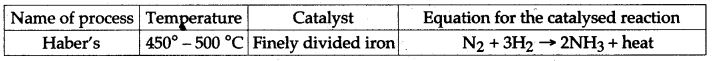### Reasoning based Questions

Question 1: Ammonia cannot be obtained in laboratory from ammonium nitrate and sodium hydroxide. Why?
Answer: This is because ammonium nitrate on heating decomposes explosively with the formation of nitrous oxide and water.

Question 2: Why ammonia gas is not collected over water ?
Answer: Ammonia gas Is not collected over water because it is highly soluble in water.

Question 3: Ammonia cannot be dried by bubbling through concentrated sulphuric acid. Why ?
Answer: Ammonia gas cannot be dried by bubbling through concentrated sulphuric acids as it reacts with sulphuric acid to form ammonium sulphate.
2NH3 + H2SO4   →    (NH4)2SO4

Question 4: Quick lime is the only drying agent used for drying ammonia gas. Why ?
Answer: Quick lime is basic in nature and hence does not react with ammonia, which is also basic in nature, whereas all other drying agents are acidic in nature and hence react with ammonia.Question 5: Aqueous solution of ammonia gives a pungent smell. Why ?
Answer: Aqueous solution of ammonia gives a pungent smell because it is produced due to bacterial decomposition of urea (NH2CONH2), present in urine.

Question 6: Ammonia solution is used as laboratory reagent to identify metal ions. Why ?
Answer: Ammonium hydroxide can precipitate insoluble hydroxide of metals from their salt solutions.
Thus, it is used in analytical analysis of salts in laboratory.

Question 7: Aqueous solution of ammonia turns red litmus blue. Give reason.
Answer: This is because ammonia on dissolving in water furnishes ammonium (NH+) ions and hydroxyl (OH) ions. The presence of OH ions in the solution turns red litmus blue.
NH3 + H2O  ⇌   NH4+ + OH

Question 8: Ammonia solution is used to remove oil and grease stains from clothes. Give reason.
Answer: When ammonium chloride is heated and rubbed with the metal, the ammonia formed removes grease, oil, etc. It emulsifies fats, grease, etc.The hydrogen chloride formed removes the oxides of metals and thus cleans the metal surfaces before soldering, tinning, etc.

Question 9: Liquid ammonia is used as refrigerant in ice plants. Give reason.
Answer: Liquid ammonia is a refrigerant as it has high latent heat of vaporisation equal to 5700 cals/mole and a low boiling point. When liquid ammonia evaporates, it takes large amount of heat from surroundings, resulting in the fall in temperature.Question 10: During the manufacture of nitric acid by Ostwald’s process excess of oxygen is taken. Give reason.
Answer: Excess of oxygen is taken because each and every step requires oxygen.

Question 11: In the laboratory preparation of nitric acid, it can be obtained below 200°C or above 200°C, but the lower temperature is preferred. Why ?
Answer: The lower temperature is favoured due to the following reasons :
(i) The glass apparatus may break at higher temperature.
(ii) A significant amount of nitric acid gets decomposed at higher temperature.
(iii) At higher temperature, potassium or sodium sulphate are formed, which cannot be easily removed from the apparatus.

Question 12: Cone. HCl is not used in place of cone. H2SO4 to prepare nitric acid from KNO3. Why ?
Answer: Cone. HCl is not used in place of cone. H2SO4 for the preparation of cone. HNO3 from KNO3 because cone. HCl is volatile and, hence, nitric acid vapours will carry HCl vapours. The property of cone. H2SO4 used in the preparation of cone. HNO3 is its lower volatility.

Question 13: Only all-glass apparatus should be used for the preparation of nitric acid. Why ?
Answer: Nitric acid is highly corrosive and hence destroys rubber and cork of the apparatus.

Question 14: Pure nitric acid take on a yellowish-brown colour when exposed to light. Why ?
Answer: When pure HNO3 is exposed to light it decomposes to give a reddish brown NO2 gas which dissolves in undecomposed nitric acid to give yellowish brown colour.

Question 15: Concentrated nitric acid fumes when kept open. Why ?
Answer: Concentrated nitric acid fumes when kept open because it contains considerable amount of nitrogen dioxide which escapes when exposed.

Question 16: Nitric acid stains the skin yellow. Give reason.
Answer: Dilute nitric acid reacts with the proteins of the skin and forms a yellow compound called xanthoproteic acid. Hence, the skin becomes yellow. Cone, nitric acid causes blisters on the skin and is highly corrosive.

Question 17: Iron is rendered passive with fuming nitric acid. Why ?
Answer: This is because of the formation of reddish brown layer of iron oxide which prevents its further reaction with the acid.

Question 18: In Haber’s process before the gases are allowed to enter the oxidation chamber, the gases are sufficiently cooled. Why ?
Answer: The gases are cooled so that the complete oxidation of nitric oxide takes place or the rate of forward reaction increases or to minimize the decomposition of nitrogen dioxide.

Question 19: The mixture of nitrogen and hydrogen gases entering the catalyst chamber must be pure. Why?
Answer: The mixture of nitrogen and hydrogen gases entering the catalyst chamber must be pure, because the presence of carbon dioxide, carbon monoxide and traces of sulphur compound poison the catalyst. Therefore, the removal of these catalyst poison from nitrogen and hydrogen is very essential.

Question 20: Aluminium does not react with nitric acid of any concentration. Why ?
Answer: Aluminum metal is not attacked by nitric acid of any concentration because of the thin and unreactive protective layer of aluminum oxide formed on the metallic surface due to the reaction of aluminium metal with oxygen of air.

Question 21: Nitrogen obtained from air is more dense as compared to nitrogen obtained from chemicals. Why ?
Answer: Nitrogen obtained from air contains traces of inert gases and therefore, it is more dense as compared to chemical nitrogen.

Question 22: Burning magnesium continues to-burn in nitric oxide, while burning sulphur is extinguished. Why ?
Answer: The heat produced by burning of magnesium is quite sufficient to decompose nitric oxide into nitrogen and oxygen. The oxygen so obtained supports combustion, but the burning of sulphur does not decompose nitric oxide hence it is extinguished.

Question 23: During a thunder storm, the rain water contains traces of nitric acid. Why ?
Answer: Nitrogen present in atmosphere combines with oxygen to form nitric oxide in the presence of electric spark due to lightening produced from a thunderstorm. Nitric oxide is further oxidized to nitrogen dioxide. Nitrogen dioxide dissolves in water in the presence of oxygen of air to form nitric acid.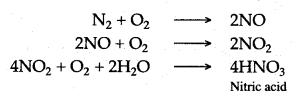Question 24: Colourless nitric oxide forms brown fumes in air. Give reason.
Answer: Nitric oxide is oxidized by the oxygen of air to form brown coloured nitrogen dioxide :Question 25: Nitric oxide gives a black colour with ferrous sulphate solution. Why ?
Answer: Nitric oxide gives a black colour solution, when it is passed through ferrous sulphate solution because nitric oxide combines with ferrous sulphate to form a black coloured complex compound known as “Nitroso ferrous sulphate”.Question 26: Nitrous oxide supports combustion more vigorously than air does. Give reason.
Answer: The percentage of oxygen present in nitrous oxide is more than air and it readily decomposes to form oxygen, which supports combustion. Nitrous oxide contains 36-37% of available oxygen, while air contains 21% of oxygen.

### Chemical Tests

Question:
1. Dilute hydrochloric acid and dilute nitric acid.
2. Ammonium ion and hydroxide ion.
3. Nitric oxide gas and nitrous oxide gas.
4. Dilute hydrochloric acid and dilute sulphuric acid.
5. Oxygen gas and nitrous oxide gas.
1. Silver nitrate solution when added to dil. hydrochloric acid will give a white ppt. and when added to dil. nitric acid no change will be observed.
2. When iron (II) sulphate solution is added to ammonium ion, dirty gree precipitate is obtained. No change will be observed with hydroxide ion.
3.If reddish brown fumes are produced in the atmosphere of oxygen then the given sample of gas is nitric oxide and if no brown fumes are produced and the mixture remains colourless, then the gas is nitrous oxide.
4. Dilute sulphuri? acid will give a white precipitate of barium sulphate with barium chloride solution whereas no visible reaction occurs with dilute hydrochloric acid.
5. Carbon (red hot) burns in oxygen to form carbon dioxide gas only, but in nitrous oxide it forms carbon dioxide gas and nitrogen.

### Balancing/Writing the Chemical Equations

Question 1: Write balanced chemcial equation for the following :
1. Reaction of Hydrogen chloride with Ammonia.
2. The preparation of Ammonia from Ammonium chloride and Calcium hydroxide.
3. Magnesium heated in nitrogen.
4. Action of concentrated nitric acid an copper.
5. Action of heat on silver nitrate.
6. Reaction of nitric acid with sodium bicarbonate.
7. Laboratory preparation of nitric acid.
8. Chlorine reacts with excess of ammonia.
9. Ferric hydroxide reacts with nitric acid.
10. Action of heat on Ammonium nitrate.
11. Dilute nitric acid producing carbon dioxide.
12. Reaction between copper and concentrated nitric acid.
13. Burning of ammonia in oxygen.
14. Catalytic oxidation of ammonia.
15. Action of heat on ammonium chloride
16. Copper and concentrated nitric acid.
17. Copper oxide and dilute nitric acid.
18. Action of cone. HNO3 on Sulphur.
19. Action of coftc. HNO3 on Phosphorus.
20. Action of cone. HNO3 on Carbon.
21. Action of cone. HNO3 on Iodine.
22. Dilute nitric add and copper.
24. Ammonia with copper oxide.
25. Ammonia with excess chlorine.
26. Action of water in magnesium nitride.
27. Burning of ammonia in oxygen.
28. Catalytic oxidationof ammmonia.
29. Aluminium nitride and water.
30. Potassium nitrate find concentrated sulphuric acid.
31. Concentrated nitric add and copper.
32. Action of heat on ammonium nitrate.
33. Nitrogen monoxide and oxygen.
34. Dilute nitric add and sodium carbonate.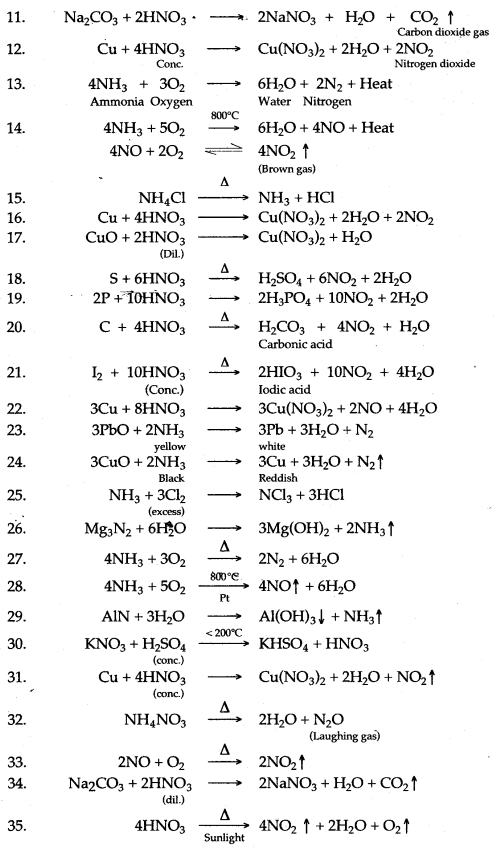Question 2: Complete and balance the following equations: